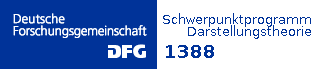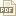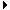﻿ DFG-Schwerpunktsprogramm Darstellungstheorie 1388Startseite
DFG- Antrag
Gäste
Projekte
Projekte (abg.)
Publikationen
Stellen
Stellenarchiv
Terminübersicht
Archiv

Publikationen
 A | B | C| D | E | F | G | H | I | J | K | L | M | N | O | P | Q | R | S | T | U | V | W | X | Y | Z

Agricola, Ilka :
• Manifolds with vectorial torsion• Quaternionic Heisenberg groups as naturally reductive homogeneous spaces• Spinorial description of SU(3)- and G2-manifolds• The classification of naturally reductive homogeneous spaces in dimensions n≤6• A note on generalized Dirac eigenvalues for split holonomy and torsion• Cones of G manifolds and Killing spinors with skew torsion• Sp(3) structures on 14-dimensional manifolds• Einstein manifolds with skew torsion• Twistorial eigenvalue estimates for generalized Dirac operators with torsionBackhaus, Teodor :
• The PBW filtration and convex polytopes in type $\tt B$• The degree of the Hilbert-Poincaré polynomial of PBW-graded modules• PBW filtration: Feigin-Fourier-Littelmann modules via Hasse diagramsBastian, Janine :
• Mutation classes of \tilde{A}_n-quivers and derived equivalence classification of cluster tilted algebras of type \tilde{A}_n• Counting the number of elements in the mutation classes of $\tilde{A}_n$-quivers• Towards derived equivalence classification of the cluster-tilted algebras of Dynkin type D• Derived equivalence classification of the cluster-tilted algebras of Dynkin type EBliem, Thomas :
• Chopped and sliced cones and representations of Kac-Moody algebras• Weight multiplicities for so5(C)• Lattice Point Generating Functions and Symmetric Cones• The number of flags in finite vector spaces: Asymptotic normality and Mahonian statistics• On the law of large numbers for Demazure modules of sl2hat• Gelfand-Tsetlin polytopes and Feigin-Fourier-Littelmann polytopes as marked poset polytopes• Expected degree of weights in Demazure modules of $\hat{sl}_2$• Towards computing vector partition functions by iterated partial fraction decompositionBlume, Mark :
• Toric orbifolds associated to Cartan matrices• The functor of toric varieties associated with Weyl chambers and Losev-Manin moduli spaces• On generalisations of Losev-Manin moduli spaces for classical root systemsBongartz, Klaus :
• Indecomposables live in all smaller lengths• On minimal disjoint degenerations of modules over tame path algebrasBossinger, Lara :
• The degree of the Hilbert-Poincaré polynomial of PBW-graded modulesBrunat, Olivier :
• On equivariant bijections relative to the defining characteristic• Counting p'-characters in finite reductive groupsBois, Jean-Marie:
• Weyl groups for non-classical restricted Lie algebras and the Chevalley restriction theorem• On maximal subfields of enveloping skewfields in prime characteristicsBürgisser, Peter :
• On a Problem Posed by Steve Smale• An overview of mathematical issues arising in the Geometric complexity theory approach to VP v.s. VNP• Coverage Processes on Spheres and Condition Numbers for Linear Programming• Even Partitions in Plethysms• Deciding Positivity of Littlewood-Richardson Coefficients• Explicit Lower Bounds via Geometric Complexity Theory• An overview of mathematical issues arising in the geometric complexity• Nonvanishing of Kronecker coefficients for rectangular shapes• Geometric Complexity Theory and Tensor Rank• Permanent versus determinant: not via saturations of monoids of representations• Binary determinantal complexity• Fundamental invariants of orbit closures• Membership in moment polytopes is in NP and coNPCerulli Irelli, Giovanni :
• Degenerate flag varieties and Schubert varieties: a characteristic free approach• Quiver Grassmannians and degenerate flag varieties• Desingularization of quiver Grassmannians for Dynkin quivers• Homological approach to the Hernandez-Leclerc construction and quiver varieties• Linear independence of cluster monomials for skew-symmetric cluster algebras• Caldero-Chapoton Algebras• Degenerate flag varieties of type A and C are Schubert varieties• Quivers with potentials associated to triangulated surfaces, Part III: tagged triangulations and cluster monomialsChen, Bo :
• The Gabriel-Roiter measure for $\widetilde{\mathbb{A}}_n$ IIChristandl, Matthias :
• Nonvanishing of Kronecker coefficients for rectangular shapes• Even Partitions in PlethysmsCupit-Foutou, Stephanie :
• Wonderful Varieties: A geometrical realization• Invariant Hilbert schemes and Wonderful varietiesDell'Ambrogio, Ivo :
• On the derived category of a graded commutative noetherian ring• Even more spectra: tensor triangular comparison maps via graded commutative 2-rings• Restriction to finite-index subgroups as étale extensions in topology, KK-theory and geometry• Grothendieck-Neeman duality and the Wirthmüller isomorphism• Gorenstein homological algebra and universal coefficient theorems• Affine weakly regular tensor triangulated categoriesDesczyk, Christian :
• The degree of the Hilbert-Poincaré polynomial of PBW-graded modules• PBW filtration: Feigin-Fourier-Littelmann modules via Hasse diagramsDipper, Richard :
• Quantized mixed tensor space and Schur-Weyl duality I• Quantized mixed tensor space and Schur-Weyl duality IIEbeling, Wolfgang :
• Multi-variable Poincaré series associated with Newton diagrams• Poincaré series and Coxeter functors for Fuchsian singularitiesEhrig, Michael :
• MV-Polytopes via affine buildingsErnst, Stefan :
• Iterative differential embedding problems in positive characteristic• 1 | 2 | 3 | 4 | Nächste| Impressum |

News

• Die Vortragsfolien der Vorträge auf der Schwerpunkttagung 2015 finden sie hier.
• Conference Volume hier.